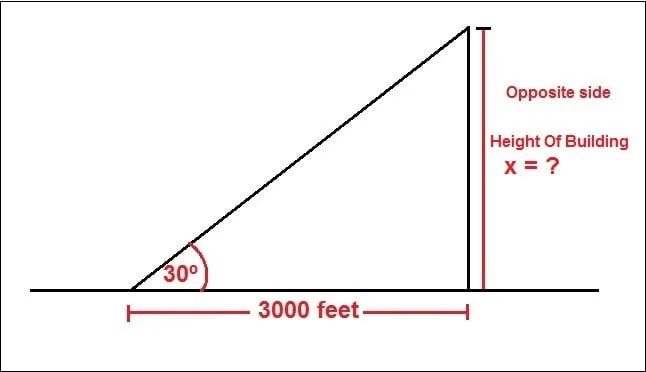# BlogSometimes we may need to find out the height of a building before or after construction. There are several methods for calculating the height of a building. In this article, I will use trigonometry method for calculating the height of the building. This is the simplest method. You can use this method to find out the height of any objects such as a tower, water tank, tree, lighthouse etc,

Required Data:

Distance and angle (as shown in fig).

Given:

Angle = θ = 30º

Distance = d = 3000 feet.Procedure:

We know,

Tangent = The ratio of the opposite side to the adjacent side.

Which means tanθ = Opposite side/Adjacent side

here θ = 30º

So tan30º = Opposite side/Adjacent side = x/d = x/3000

x = tan30º x 3000 = 0.577 x 3000 = 1732 feet.

∴ The height of the building is 1732 feet.